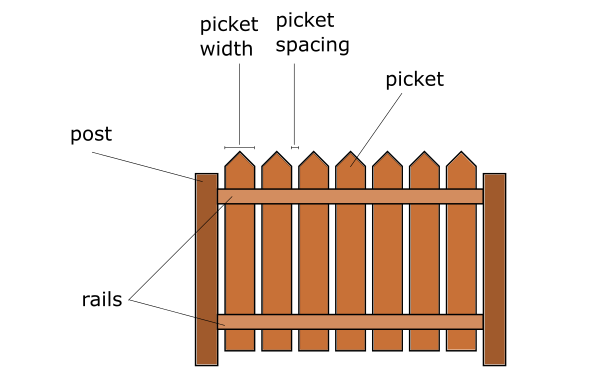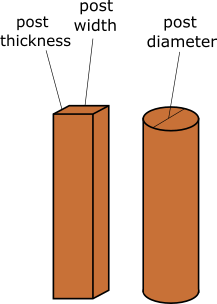# Fence Estimate Calculator

Created by Gabriela Diaz
Reviewed by Luis Hoyos
Last updated: Jun 05, 2023

There's no need to keep guessing; with Omni's fence estimate calculator, you can get a good estimation of how much fencing material you need to build your fence.

Renovation projects can be stressful situations, especially if you don't know where to start or are working on a limited budget. Knowing beforehand how many posts, pickets, and concrete you'll need to build a fence can help you plan, make smart money decisions, and save money and time!

Continue reading to learn how much material you'll need to complete the fence, and also figure out:

• How to use this tool;

• How many feet of fence for a 1/2 acre field you need; and

• How much concrete for a 4x4 fence post you need.

## Using the fence estimate calculatorThe fence estimate calculator can be a great tool to help you save time and money when planning to build a fence. It allows you to quickly estimate the amount of material you need. Let's go through how to use this tool!

But before we begin, you need to know the dimensions of your yard or the total length of the fence you want to build. If you don't have these measurements just yet, it's time to go outside and measure. Don't worry; we'll be here waiting for you 😉 If you already have your measurements, let's move on to see how to use this tool:

1. Begin by entering the Fence length and Post space. This last one is set as 2.5 meters as default, but typically, the post space is anywhere between 6 and 8 feet; or 2 and 5 meters, depending on your preference.

2. With this information, the calculator will display the Number of posts and Number of sections.

3. Next, input the Fence height, and the calculator will show the Post length. Notice that at least 1/3 of every post should be buried in the ground and secured with concrete.

4. Now indicate the Picket width and Picket spacing. Pickets' width is often between 3 1/2", 5 1/2", or up to 11 1/2". Typically, picket spacing is 2 1/2", but it can also vary anywhere between 1" to 6".

5. The calculator will indicate the number of pickets.

6. Finally, you can determine the volume of concrete you need for post footing. For this, you need to enter either the diameter of your post for cylindrical posts or the width and thickness of the post for cuboid posts.Did you find this tool useful? If you like to keep learning about fence calculations, we invite you to take a look at our other related tools:

## FAQ

### How much concrete for a 4x4 fence post do I need?

You need 4608 in3 of concrete for one 4x4-inch post in a 6 ft tall fence. To obtain this value:

1. Use the following formula:

volume_of_concrete = (hole_volume - post_volume) × number_of_posts

2. To obtain the hole_volume:

hole_volume = (3×post_width) × (3×post_thickness) × (1/2×fence_height)

hole_volume = (3×4 in) × (3×4 in) × (1/2×6 ft) = 5184 in3

3. Determine the post_volume:

post_volume = post_width × post_thickness × (1/2×fence_height)

hole_volume = 4 in × 4 in × (1/2×6 ft) = 576 in3

4. Substitute these results in the volume_of_concrete formula:

volume_of_concrete = (5184 in3 - 576 in3) × 1 = 4608 in3

### How many feet of fence for a 1/2 acre do I need?

You need a minimum of 590.32 ft of fence for a 1/2 acre. Since acres are a measure of area, the exact amount of fence you need depends on the shape of the land:

• For a squared fence, you need 590.32 ft. This corresponds to a 147.58 ft × 147.58 ft area.

• For a rectangular fence with a length that's twice the width, you need 626.13 ft. This is a 104.35 ft × 208.71 ft area.

### How do I calculate the number of fence posts needed?

To calculate the number of fence posts that you need:

1. Use the following formula:

Number of posts = (fence length / post spacing) + 1

2. Typical post spacing is between 6 and 8 ft, or 2 to 3 m.

3. Make sure to convert all lengths to the same unit.

4. Round the result to the next whole number.

For example, for a 300 ft fence with 7 ft post spacing:

Number of posts = (300 ft / 7 ft) + 1
Number of posts = 43.86
Number of posts ≈ 44

Gabriela DiazNumber and length of posts needed
Fence length
ft
Post space
ft
Number of posts
Number of sections
Fence height
ft
Post length
ft
Number of rails needed
Rails per section
Number of rails
Number of pickets needed
Picket width
in
Picket spacing
in
Number of pickets
Concrete for post footing
Post shape
cuboid
Post width
in
Post thickness
in
Concrete volume
cu yd
Visit the cement calculator to determine how much cement, sand, gravel, water, or money you'll need for this concrete volume.
People also viewed…

Addiction calculator tells you how much shorter your life would be if you were addicted to alcohol, cigarettes, cocaine, methamphetamine, methadone, or heroin.

### Air changes per hour

With the air changes per hour calculator (ACH) you can determine how often your air device exchanges the air in a room.

### Heat loss

Use the heat loss calculator to estimate the power of a heater needed to keep your room at a comfortable temperature.

### Sleep

The sleep calculator can help you determine when you should go to bed to wake up happy and refreshed.